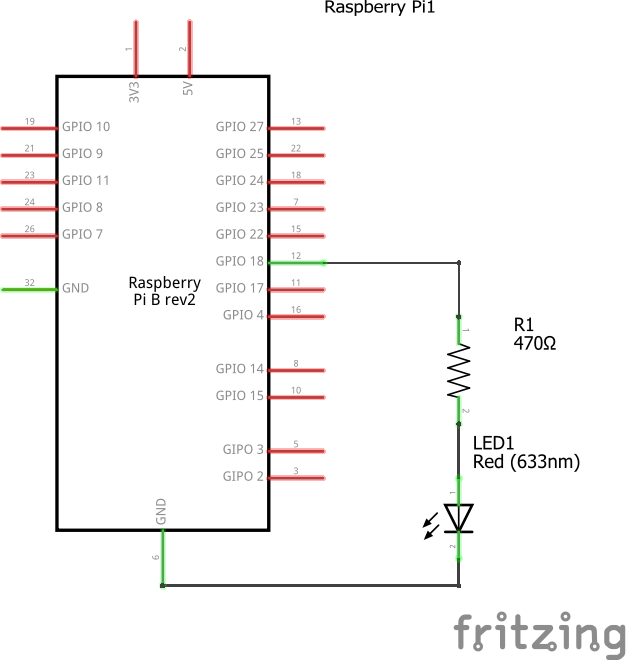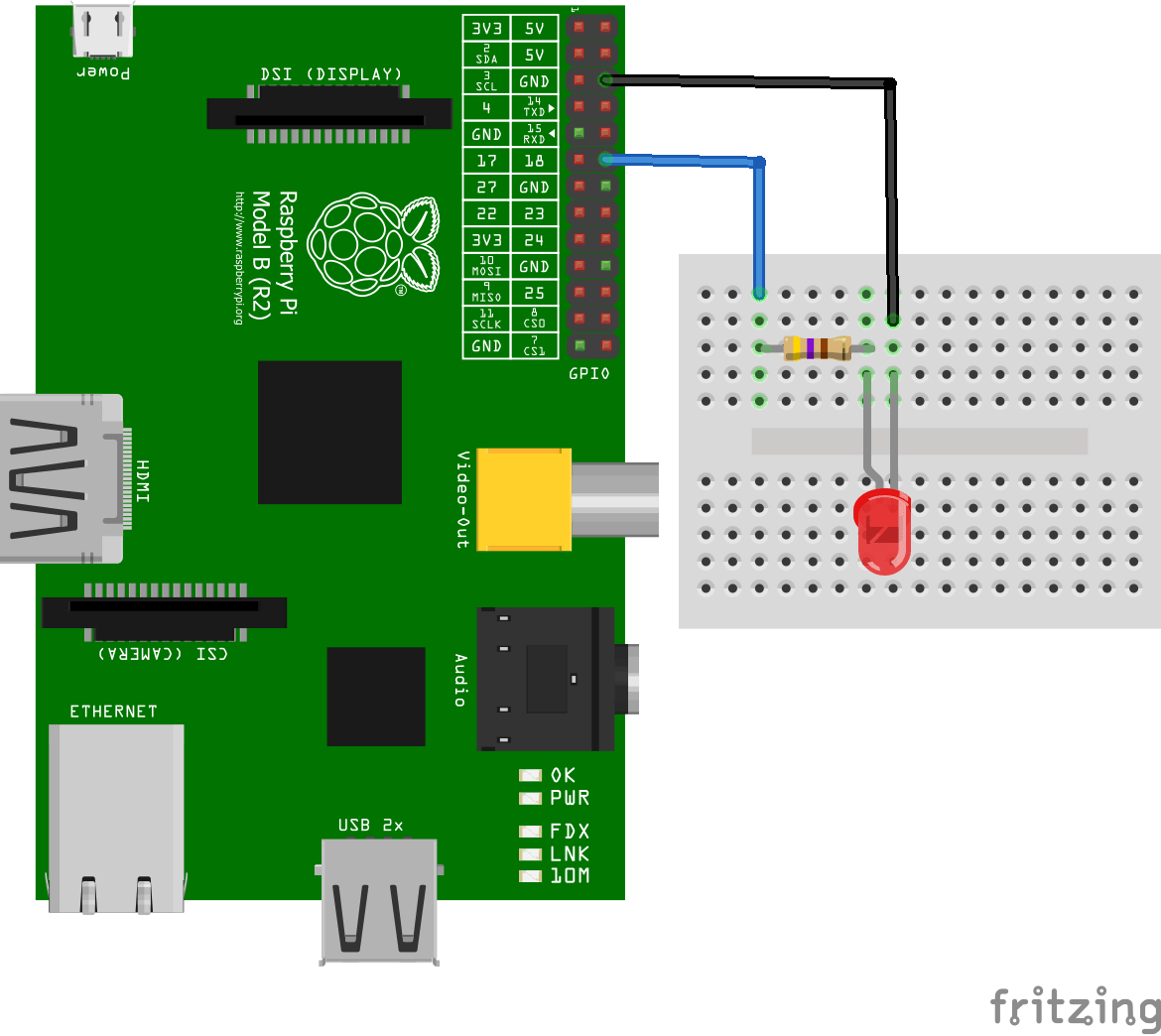Home Learning Controlling the Brightness of an LED

# Controlling the Brightness of an LED

In this example we will show you how to control the brightness of an LED connected . The RPi.GPIO library has a PWM feature that allows you to control the power to an LED and its brightness, we will use this in this example

Schematic

Fairly simple, simply connect an LED like thisThis is a breadboard layoutCode

Save the following as pwm.py

 Source code```import RPi.GPIO as GPIO

#pin 12 on GPIO header
led_pin = 18
GPIO.setmode(GPIO.BCM)
GPIO.setup(led_pin, GPIO.OUT)
pwm_led = GPIO.PWM(led_pin, 500)
pwm_led.start(100)

while True:
brightness = raw_input("Enter Brightness value (0 to 100):")
duty_value = int(brightness)
pwm_led.ChangeDutyCycle(duty_value)```

Testing

From a terminal run the program by typing in

sudo python pwm.py

You should now see a prompt, type in a value and press enter and observe the effect on the LED